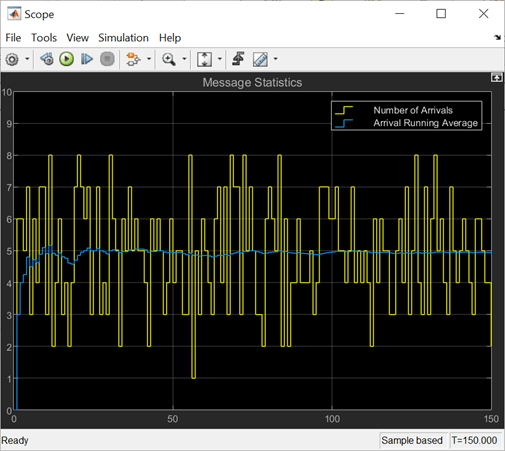# getInterfaceImpl

Set System object as message or data

## Syntax

```interface = getInterfaceImpl(obj) ```

## Description

MATLAB® `interface = getInterfaceImpl(obj)` specifies the inputs and outputs of a System block as either data or a message.

### Run-Time Details

`getInterfaceImpl` is part of the `matlab.System` class and is called before the `setupImpl` method.

### Method Authoring Tips

You must set `Access = protected` for this method.

You cannot modify any properties in this method.

## Input Arguments

expand all

System object handle used to access properties, states, and methods specific to the object. If your `getInterfaceImpl` method does not use the object, you can replace this input with `~`.

## Output Arguments

expand all

MATLAB System block interface, returned as a scalar or vector of `matlab.system.interface.*` objects. Use `matlab.system.interface.Input`(`signalName`,`signalType`) to define input signal types. Use `matlab.system.interface.Output`(`signalName`,`signalType`) to define output signal types.

• `signalName` – Defines the name of the port. Represented by a character array.

• `signalType` – Defines the type of port. The value is either `matlab.system.interface.Data` or `matlab.system.interface.Message`.

## Examples

expand all

This example shows how to send, receive, and process messages using the MATLAB System block. Use System objects to author blocks to model custom behavior to send and receive messages and manipulate the message payload.

Open the model of the Message Send and Receive system. This model contains a random number generator as a data source. Based on that data, the Message Sender sends a message with a sine wave payload to a queue block. The queue block stores the messages, and the Message Receiver converts the message back to data.

```open_system('slexMessageArrivalExample'); ```Use `getInterfaceImpl` System Object API to Specify Message Ports

`getInterfaceImpl` is used in this example to specify the input and output message ports in the MATLAB System block for both the Message Sender and the Message Receiver. For the Message Sender, `getInterfaceImpl` is used to define the output of the MATLAB System block as a message. This action prompts the System object to create a message output. For the Message Receiver, `getInterfaceImpl` is used to define the input of the System object as a message and the output as data.

```%Function to send messages function interface = getInterfaceImpl(~) import matlab.system.interface.*; interface = Output("Out1", Message); end ```
```%Function to receive messages and output as data function interface = getInterfaceImpl(obj) import matlab.system.interface.*; interface = [Input("In1", Message), ... Output("Out1", Data), Output("out2", Data)]; end ```

Set the Propagators and Sample time

The following four propagators need to be set in the Message Sender: `getOutputSizeImpl`, `getOutputDataTypeImpl`, `isOutputComplexImpl`, `isOutputFixedSizeImpl`.

In this example, the message queue has a maximum capacity of 16 messages. The random number generator has a sample time of 0.1. The receiver has a sample time of 1. The Message Receiver sample time is set in the MATLAB System block using the `getSampleTimeImpl` API.

```function sts = getSampleTimeImpl(obj) sts = createSampleTime(obj,'Type','Discrete', ... 'SampleTime',obj.SampleTime); end ```

Simulate the Model and Review Results

The Scope block displays the results. These results show that as you run more simulations, the random number generator produces a number greater than zero 50% of the time, as expected.## Version History

Introduced in R2021a